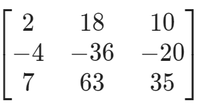GFG App
Open AppBrowser
Continue

# GATE | GATE-CS-2014-(Set-2) | Question 14

If the matrix A is such thatthen the determinant of A is equal to

(A)

0

(B)

1

(C)

2

(D)

3

Explanation:

This is a numerical answer question of gate paper, in which no options are provided, and the answer is to given by filling a numeral into a text box provided.

In the question, matrix A is given as the product of 2 matrices which are of order 3 x 1 and 1 x 3 respectively. So after multiplication of these matrices, matrix A would be a square matrix of order 3 x 3.

So, matrix A is :Now, we can observe by looking at the matrix that row 2 can be made completely zero by using row 1, this is to be done by using the row operation of matrix which here is : R2 <- R2 + 2R1 After applying above row operation in the matrix, the resultant matrix would be: 2 18 10 0 0 0 7 63 35 i.e. Row 2 has become zero now. And if a square matrix has a row or column with all its elements as 0, then its determinant is 0. ( A property of a square matrix ) Hence answer is 0. Note: Determinant is defined only for square matrices, and it is a number which encodes certain properties of a matrix, for ex: a square matrix with determinant 0 does not has its inverse matrix.

Quiz of this Question
Please comment below if you find anything wrong in the above post

My Personal Notes arrow_drop_up